Once the structure has been materialized (each bar represents a particular section of a given material), we can begin the process of calculation. So we have three possibilities:

• Menu Calculate | Iterative process
• F5 key
• Button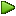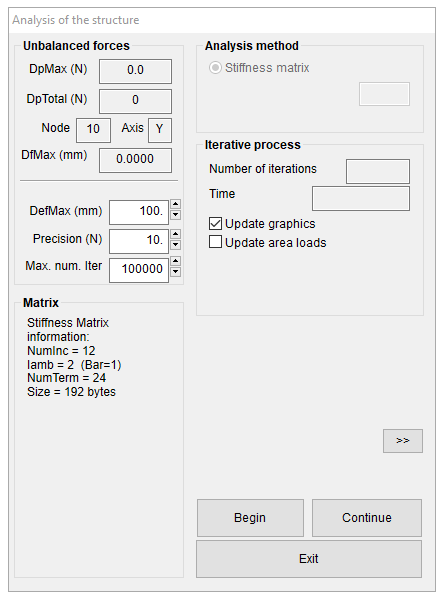With any of them you get the window that is displayed above this text. This window is the control panel that will allow you to manipulate the iterative calculation process, as we must not forget that we deal with structures of non-linear behaviour.

In this window, there are different groups of controls, enclosed in a rectangle. We will discuss each one of them:

##### Unbalanced forces

It’s informative values. They are presented by the program gives and, obviously, the user can not manipulate them:

• DpMax (N) or (kg)
Informs us of the maximum imbalance (absolute value) at a node, in any of the X,Y,Z axes. In the image the value is ……. kg
• DpTotal (N) or (kg)
The sum of all the imbalances (in absolute value) at all nodes, in any axes X,Y,Z. In the image the value is …… kg
• Node
Informs us which is the node that has generated the value DpMax
• Axis
Informs us in which axis the DpMax value has been generated
• DfMax (mm)
Informs us which of the nodes of the structure in the last iteration has the maximum displacement in any of the axs X,Y,Z. In the case of the image it is set to zero which means that the structure has not yet been displaced, i.e. the iterative process has not begun.

Then there is a separator line followed by other values that, unlike the previous, they can be modified by the user.

• DefMax (mm)
This is the maximum value of displacement in each iteration, in any of the X,Y,Z axes that you allow to the structure. This is a delicate value, because if we allow large displacement for each iteration, the structure may go more quickly to the equilibrium position, But in that case, it is easier that the process becomes unstable, and the structure completely deforms and never achieves the balance. On the other hand, if we restrict this value too much, the movement will be very slow, with what the iterative process takes very long time, but the danger of complete distortion of the structure is minimal.Empirically, it is recommended to start the process with a value of 100 mm. If the structure is very large and we think that final displacements will also be very large, we can opt for higher values of up to 1000 mm.

When we are approaching the balance, if we note that the iterative process has been stalled, we can decrease slowly (with the arrows next to the box) the value of DefMax and look at a more rapid increase of convergence(the process of finding the balance by means of iterations is called convergence).

• Precision
An iterative process tends to find the balance. But there will always be a definition of balance. Working with numeric values with many decimal digits, a perfect balance is virtually impossible to achieve. As an added absurdness, the material reality we use in calculating is far from the numerical methods we are using.
By default WinTess3 uses the value of 1 kg (10 N) as acceptable balance. i.e., if the absolute value of DpMax is equal to or less than 1 kg, we accept that the structure is in balance.
• Num. Max. Iter.
In all iterative process there is a possibility that there is no convergence for some reason. It is therefore interesting to have the possibility to stop this process at a given time. The number of iterations is very closely linked with the method of calculation. Currently the only method of calculating method for a normal user is the Matrix method, therefore the considerations we do refer to this method.
Default is the value of 100000, which is the same as saying never stop until you find the balance. However, there will be times that we want to stop the process at any given time (a common case is that you only want to do one iteration) for watching the displacement and making subsequent decisions. We also can stop the process every 5 iterations or something like that.
##### Matrix

This box informs us of certain values associated with the matrix method:

• NumInc
It is the total number of unknowns from the stiffness matrix of the structure. A typical free node of the mesh generates three unknowns: displacement in X, Y, Z. In contrast end node of a rigid bar generates six unknowns: displacement X,Y,Z and rotations X,Y,Z.
• Iamb
This value, called bandwidth, indicates the relations between the unknowns of the two nodes of a bar. If the displacement X,Y,Z of the node i of a bar are the 135,136,137 and the displacement X,Y,Z of the node j of a bar are the 314,315,316 , the bandwidth of this bar is 316-135 = 181. The maximum bandwidth of all the bars is the bandwidth of the stiffness matrix. Given that the numbers of unknowns (135, 136, …) is produced according to the numbering of the nodes, it is seen that this numbering of nodes is not trivial. When you generate a mesh, the program automatically numbers the nodes, but then, during the process of modification of the mesh, the inclusion of external elements, such as arches, masts, etc. , this numbering system may prove to be inappropriate.For this reason, the program has a menu Nodes | Renumber all nodes that reorganizes the numbering of nodes. In case of doubt, it is interesting to run this menu and see if the Iamb value has declined. Clearly, this will accelerate the iterative process of calculation.
• NumTerm
It is the number of terms of the stiffness matrix. It is obtained by multiplying NumInc by Iamb.
• Size
It is the size of the stiffness matrix in bytes. As the calculation is carried out with real numbers of double precision (8 bytes) the size of the array is equal to NumTerm by eight.
##### Analysis methods

Although the program has the possibility to use different analysis methods, this variety of methods is only used for research.
For normal use, all these methods have been optimized in a single one: the matrix method.
to arrive to this optimization we have used these other methods

• Dynamic relaxation
Very popular iterative method in nonlinear analysis based on simulating processes of particles movements with accelerations and springs with fictitious masses located at the nodes (http://en.wikipedia.org/wiki/Dynamic_relaxation).
• Geometric relaxation
Derived from the above, with a faster iteration process but slower convergence method, especially for small precision.
• Point to point
Slow but a didactic method in which the structure is balancing node to node, every time releasing the knot with major imbalance and balancing it (It is a method similar to Cross method for frames analysis).
##### Iterative process

There are two information values in this box:

• Number of iterations
It shows how many iterations have been carried out. When the Start button is pressed this value returns to zero.
• Time
It indicates how much time has passed in the iterative process. This value is only updated at each new iteration. When you press the Start button this value goes back to zero.

We also have some check boxes:

• Update graphics
If the box is checked, each iteration will redraw the structure in the main window, so that we can observe the process of moving.
If the box is checked, each iteration recalculates the loads on the structure, since certain actions (wind, snow, etc. ) depend on the shape of the structure.
• Update internal pressure
This box is active only for pneumatic structures where the internal pressure is not zero. If the box is checked, each iteration recalculates the internal pressure, taking into account change of the interior volume. Updated value of internal pressure appears in parentheses next to the initial internal pressure as shown below.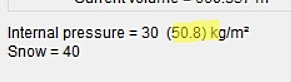We can also see the values of the current volume and the initial volume in the state of balance before applying the external actions, as shown below.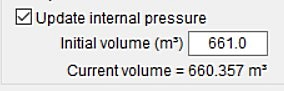• Initial volume calculated by WinTess (from the plane Z = 0 to the membrane) may not be the real initial volume. In this case we can write the value of the initial volume directly in the box.
We will have to be very careful with this change because the result can be very different.
##### Triangular elements

It is operational only for research when the program is run from the code. The options are:

• Bars
This is the calculation method in which the surface membranes are discretized in a mesh bar. At the moment it is the only operating method.
• Finite elements
This is the calculation method in which the surface membranes are discretized into triangles and analyzed using the finite element method. It is not available at the moment. (it may be soon available to all users).
##### Actions

Below the Iterative process box, we can see the actions that have been applied to the structure being analysed.

If the analysed structure is very big or complex, iterative process can take some time. If we want to control visually this process we can press button “>>” and this window is lightly enlarged.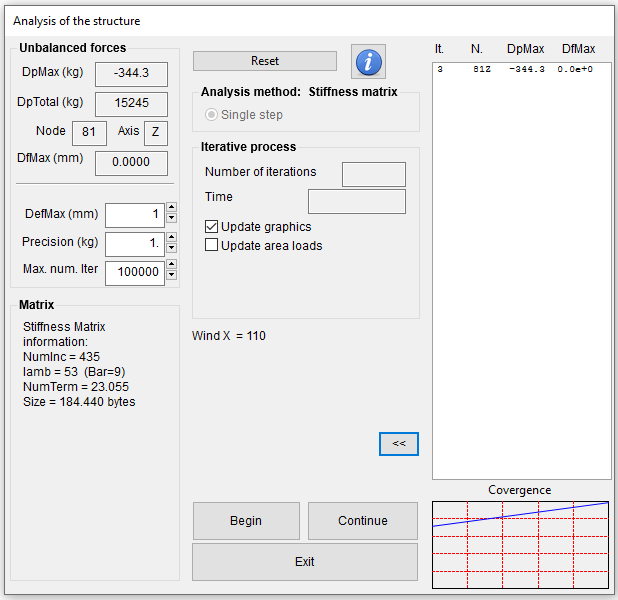In the right box we can follow the iterative process, step by step, seeing how unbalance and displacements evolve.

##### Buttons

Finally we find three buttons that start or stop processes discussed so far:

• Begin
The structure returns to the initial state and the iterative process starts again.
• Continue
Iterative process begins not from the initial form but from the position that the structure is in.
• Exit / Stop
This button can have two functions according to the text that it has. When the iterative process of calculation is stopped the text is Exit. By pressing this button closes the window for the Analysis of the structure. However, if we are in the iterative process, the button text is Stop. Pressing it stops the iterative process and we can perform any operation. After that we can start the process again, continue it or simply exit and close the window.Another way to stop analysis process consists in pressing Esc key.